﻿ Simplification of Coefficients in Two Families of Nonlinear Ordinary Differential EquationsPublications are Open
Access in this journal
Article Versions
Export Article
• Normal Style
• MLA Style
• APA Style
• Chicago Style
Original Article
Open Access Peer-reviewed

### Simplification of Coefficients in Two Families of Nonlinear Ordinary Differential Equations

Feng Qi , Bai-Ni Guo
Turkish Journal of Analysis and Number Theory. 2018, 6(4), 116-119. DOI: 10.12691/tjant-6-4-2
Received April 29, 2018; Revised June 02, 2018; Accepted July 27, 2018

### Abstract

In the paper, in terms of the Stirling numbers of the first and second kinds, by three approaches, the author derives simple, meaningful, and significant forms for coefficients in two families of nonlinear ordinary differential equations.

### 1. Motivation and Main Results

In [ 6, Theorems 2.1 and 2.2], it was established inductively and recursively that the function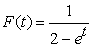(1)

satisfies the families of nonlinear ordinary differential functions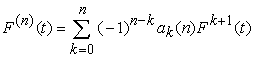(2)

and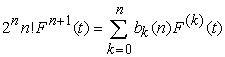(3)

for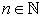, where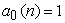,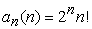,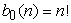,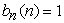,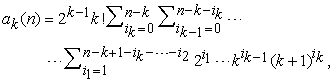(4)

and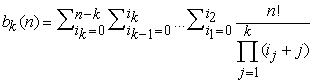(5)

for 0 < k < n.

In this paper, since

(1) the original proofs of [ 6, Theorems 2.1 and 2.2] are long and tedious,

(2) the expressions in (4) and (5) are too complex to be remembered, understood, and computed easily,

we will provide three simple and standard proofs for [ 6, Theorems 2.1 and 2.2] and, more importantly, derive simple, meaningful, and significant expressions for the quantities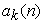and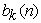.

Our main results can be stated as the following theorem.

Theorem 1. For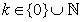, the function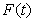defined by (1) satisfies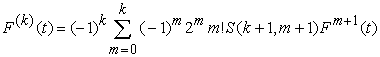(6)

and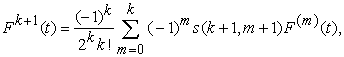(7)

where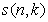and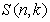stand for the Stirling numbers of the first and second kinds.

### 2. Proofs of Theorem 1

In this section, we provide three proofs for Theorem 1 as follows.

First proof. It is well known [ 1, Theorem 11.4] and [ 2, p. 139, Theorem C] that the Faàdi Bruno formula can be described in terms of the Bell polynomials of the second kind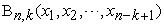by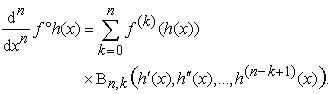(8)

The identities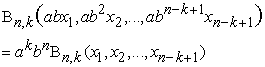(9)

and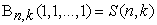(10)

for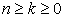and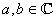can be found in [ 1,p. 412] and [ 2, p. 135]. Applying (8), (9), and (10) in sequence and denoting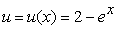yield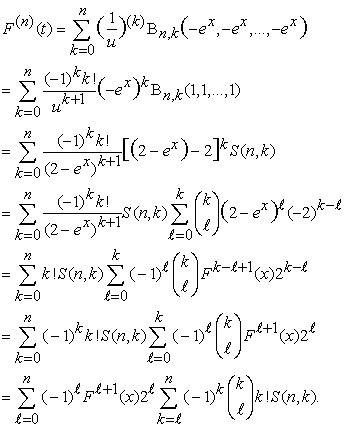Combining this with the identity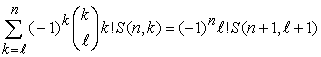(11)

in [ 24, Theorem 2.2] results in (6).

The identity (7) follows from applying [ 2, p. 213, eq. (5c)] or [ 34, p. 171, Theorem 12.1], which reads that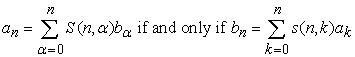for a collection of constants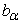and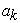independent of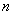, to (6). The first proof of Theorem 1 is complete.

Second proof. A sequence of polynomials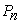of orderare called 5, 9, 22 the derivative polynomials of a function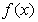if and only if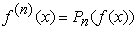for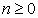. In [ 38, Theorem 1.1], it was obtained that the derivative polynomials of the function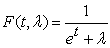can be computed by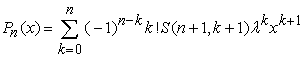(12)

for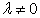and. In [ 38, Theorem 1.3], it was obtained that the nonlinear differential equations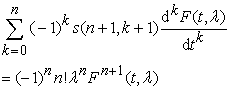(13)

have a common solutionforand. Letting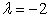in (12) and (13) gives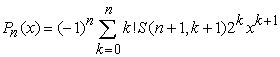and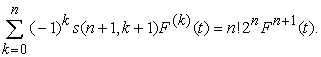The second proof of Theorem 1 is thus complete.

Third proof. By virtue of Theorem 2.1 in 3, Theorems 3.1 and 3.2 in 37, and Lemma 2.1 in 38, it follows that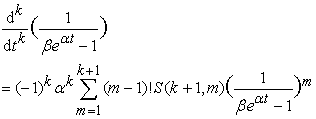(14)

and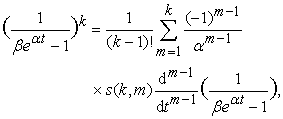(15)

where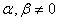are real constants,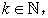either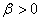and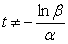or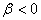and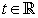. See also 4, 35, 36.

Taking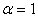and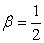in (14) and (15) leads to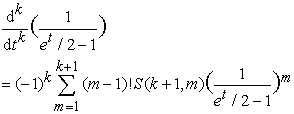and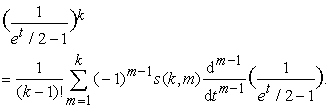These two identities can be further rearranged as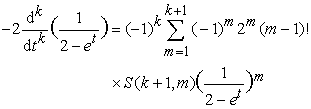and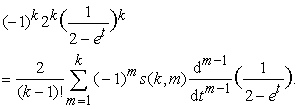The third proof of Theorem 1 is thus complete.

### 3. Remarks

Finally, we list several remarks on our main results and closely related things.

Remark 1. Comparing (2) and (3) with (6) and (7) figures out that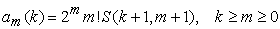and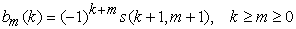which are simpler, more meaningful, and more significant than the expressions in (4) and (5).

Remark 2. In [ 34, p. 118, Eq. (9.18)], it is listed that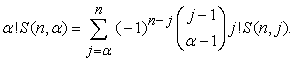This identity is different from (11) and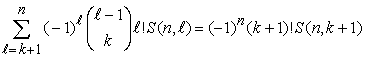(16)

for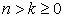in [ 24, Theorem 2.2].

Remark 3. Any one among three proofs is simpler and shorter than the one in the paper 6.

Remark 4. The motivations in the papers 3, 4, 7, 8, 10, 11, 12, 14, 15, 16, 17, 18, 19, 20, 21, 22, 23, 24, 25, 26, 27, 28, 29, 30, 31, 32, 33, 38 are same as the one in this paper.

Remark 5. This paper is a slightly modified version of the preprint 13.

### References

  C. A. Charalambides, Enumerative Combinatorics, CRC Press Series on Discrete Mathematics and its Applications. Chapman & Hall/CRC, Boca Raton, FL, 2002. In article PubMed  L. Comtet, Advanced Combinatorics: The Art of Finite and Infinite Expansions, Revised and Enlarged Edition, D. Reidel Publishing Co., 1974. In article View Article  B.-N. Guo and F. Qi, Explicit formulae for computing Euler polynomials in terms of Stirling numbers of the second kind, J. Comput. Appl. Math. 272 (2014), 251-257. In article View Article  B.-N. Guo and F. Qi, Some identities and an explicit formula for Bernoulli and Stirling numbers, J. Comput. Appl. Math. 255 (2014), 568-579. In article View Article  M. E. Hoffman, Derivative polynomials for tangent and secant, Amer. Math. Monthly 102 (1995), no. 1, 23-30. In article  G.-W. Jang and T. Kim, Some identities of ordered Bell numbers arising from differential equation, Adv. Stud. Comtemp. Math. 27 (2017), no. 3, 385-397. In article  F. Qi, A simple form for coefficients in a family of nonlinear ordinary differential equations, Adv. Appl. Math. Sci. 17 (2018), no. 8, 555-561. In article  F. Qi, A simple form for coefficients in a family of ordinary differential equations related to the generating function of the Legendre polynomials, Adv. Appl. Math. Sci. 17 (2018), in press; ResearchGate Preprint (2017). In article View Article  F. Qi, Derivatives of tangent function and tangent numbers, Appl. Math. Comput. 268 (2015), 844-858. In article View Article  F. Qi, Explicit formulas for the convolved Fibonacci numbers, ResearchGate Working Paper (2016). In article View Article  F. Qi, Notes on several families of differential equations related to the generating function for the Bernoulli numbers of the second kind, Turkish J. Anal. Number Theory 6 (2018), no. 2, 40-42. In article  F. Qi, Simple forms for coefficients in two families of ordinary differential equations, Glob. J. Math. Anal. 6 (2018), no. 1, 7-9. In article  F. Qi, Simplification of coefficients in two families of nonlinear ordinary differential equations, ResearchGate Preprint (2017). In article View Article  F. Qi, Simplifying coefficients in a family of nonlinear ordinary differential equations, Acta Comment. Univ. Tartu. Math. (2018), in press; ResearchGate Preprint (2017). In article View Article  F. Qi, Simplifying coefficients in a family of ordinary differential equations related to the generating function of the Laguerre polynomials, ResearchGate Preprint (2017). In article View Article  F. Qi, Simplifying coefficients in a family of ordinary differential equations related to the generating function of the Mittag-Leffler polynomials, ResearchGate Preprint (2017). In article View Article  F. Qi, Simplifying coefficients in differential equations related to generating functions of reverse Bessel and par- tially degenerate Bell polynomials, Bol. Soc. Paran. Mat. (2019), in press; ResearchGate Preprint (2017). In article View Article  F. Qi and B.-N. Guo, A diagonal recurrence relation for the Stirling numbers of the first kind, Appl. Anal. Discrete Math. 12 (2018), no. 1, 153-165. In article  F. Qi and B.-N. Guo, Explicit formulas and recurrence relations for higher order Eulerian polynomials, Indag. Math. 28 (2017), no. 4, 884-891. In article  F. Qi and B.-N. Guo, Explicit formulas for derangement numbers and their generating function, J. Nonlinear Funct. Anal. 2016, Article ID 45, 10 pages. In article  F. Qi and B.-N. Guo, Some properties of the Hermite polynomials and their squares and generating functions, Preprints 2016, 2016110145, 14 pages. In article View Article  F. Qi and B.-N. Guo, Viewing some ordinary differential equations from the angle of derivative polynomials, Iran. J. Math. Sci. Inform. 14 (2019), no. 2, in press; Preprints 2016, 2016100043, 12 pages. In article View Article  F. Qi, D. Lim, and B.-N. Guo, Explicit formulas and identities for the Bell polynomials and a sequence of polynomials applied to differential equations, Rev. R. Acad. Cienc. Exactas Fís. Nat. Ser. A Mat. RACSAM (2018), in press. In article View Article  F. Qi, D. Lim, and B.-N. Guo, Some identities related to Eulerian polynomials and involving the Stirling numbers, Appl. Anal. Discrete Math. 12 (2018), no. 2, in press. In article View Article  F. Qi, D.-W. Niu, and B.-N. Guo, Simplification of coefficients in differential equations associated with higher order Frobenius-Euler numbers, Preprints 2017, 2017080017, 7 pages. In article View Article  F. Qi, D.-W. Niu, and B.-N. Guo, Simplifying coefficients in differential equations associated with higher or- der Bernoulli numbers of the second kind, Preprints 2017, 2017080026, 6 pages. In article View Article  F. Qi, D.-W. Niu, and B.-N. Guo, Some identities for a sequence of unnamed polynomials connected with the Bell polynomials, Rev. R. Acad. Cienc. Exactas Fís. Nat. Ser. A Math. RACSAM 112 (2018), in press. In article View Article  F. Qi, X.-L. Qin, and Y.-H. Yao, The generating funtion of the Catalan numbers and lower triangular integer matrices, Preprints 2017, 2017110120, 12 pages. In article View Article  F. Qi, J.-L. Wang, and B.-N. Guo, Notes on a family of inhomogeneous linear ordinary differential equations, Adv. Appl. Math. Sci. 17 (2018), no. 4, 361-368. In article  F. Qi, J.-L. Wang, and B.-N. Guo, Simplifying and finding nonlinear ordinary differential equations, ResearchGate Working Paper (2017). In article  F. Qi, J.-L. Wang, and B.-N. Guo, Simplifying differential equations concerning degenerate Bernoulli and Euler numbers, Trans. A. Razmadze Math. Inst. 172 (2018), no. 1, 90-94. In article  F. Qi and J.-L. Zhao, Some properties of the Bernoulli numbers of the second kind and their generating function, Bull. Korean Math. Soc. 55 (2018). In article  F. Qi, Q. Zou, and B.-N. Guo, Some identities and a matrix inverse related to the Chebyshev polynomials of the second kind and the Catalan numbers, Preprints 2017, 2017030209, 25 pages. In article View Article  J. Quaintance and H. W. Gould, Combinatorial Identities for Stirling Numbers, the unpublished notes of H. W. Gould, with a foreword by George E. Andrews, World Scientific Publishing Co. Pte. Ltd., Singapore, 2016. In article  C.-F. Wei and B.-N. Guo, Complete monotonicity of functions connected with the exponential function and derivatives, Abstr. Appl. Anal. 2014 (2014), Article ID 851213, 5 pages. In article  A.-M. Xu and G.-D. Cen, Closed formulas for computing higher-order derivatives of functions involving exponential functions, Appl. Math. Comput. 270 (2015), 136-141. In article View Article  A.-M. Xu and Z.-D. Cen, Some identities involving exponential functions and Stirling numbers and applications, J. Comput. Appl. Math. 260 (2014), 201-207. In article View Article  J.-L. Zhao, J.-L. Wang, and F. Qi, Derivative polynomials of a function related to the Apostol-Euler and Frobenius-Euler numbers, J. Nonlinear Sci. Appl. 10 (2017), no. 4, 1345-1349. In articleThis work is licensed under a Creative Commons Attribution 4.0 International License. To view a copy of this license, visit http://creativecommons.org/licenses/by/4.0/